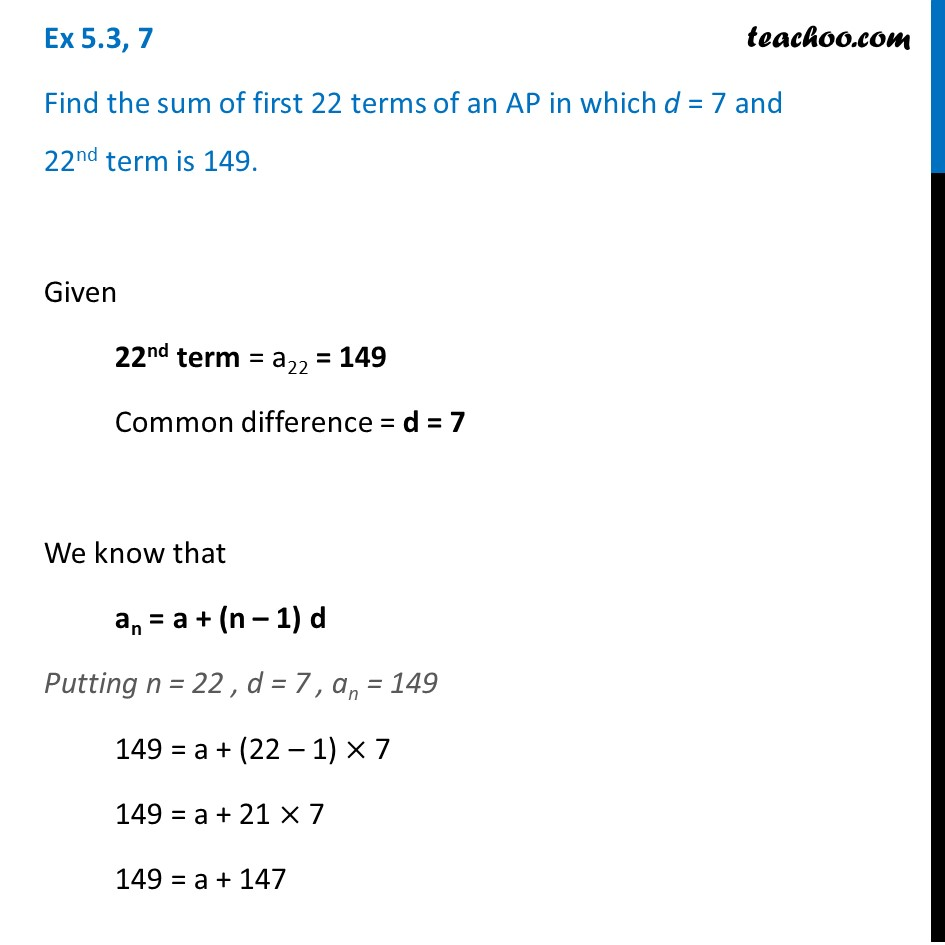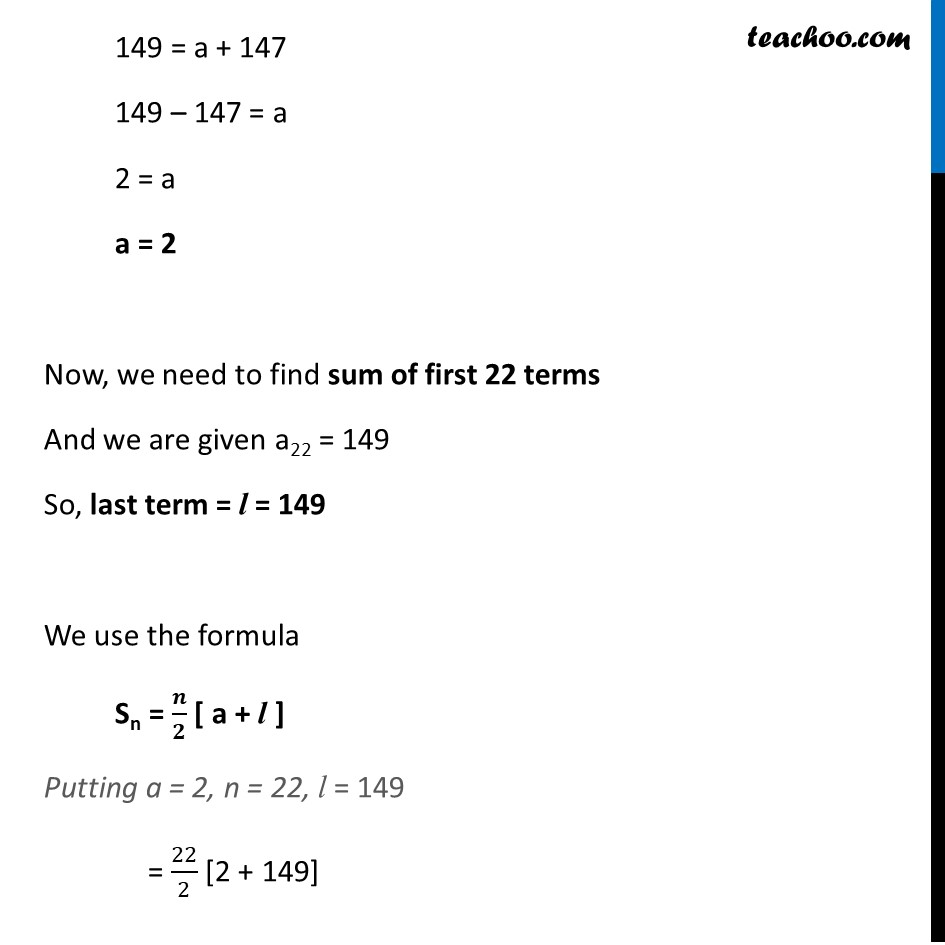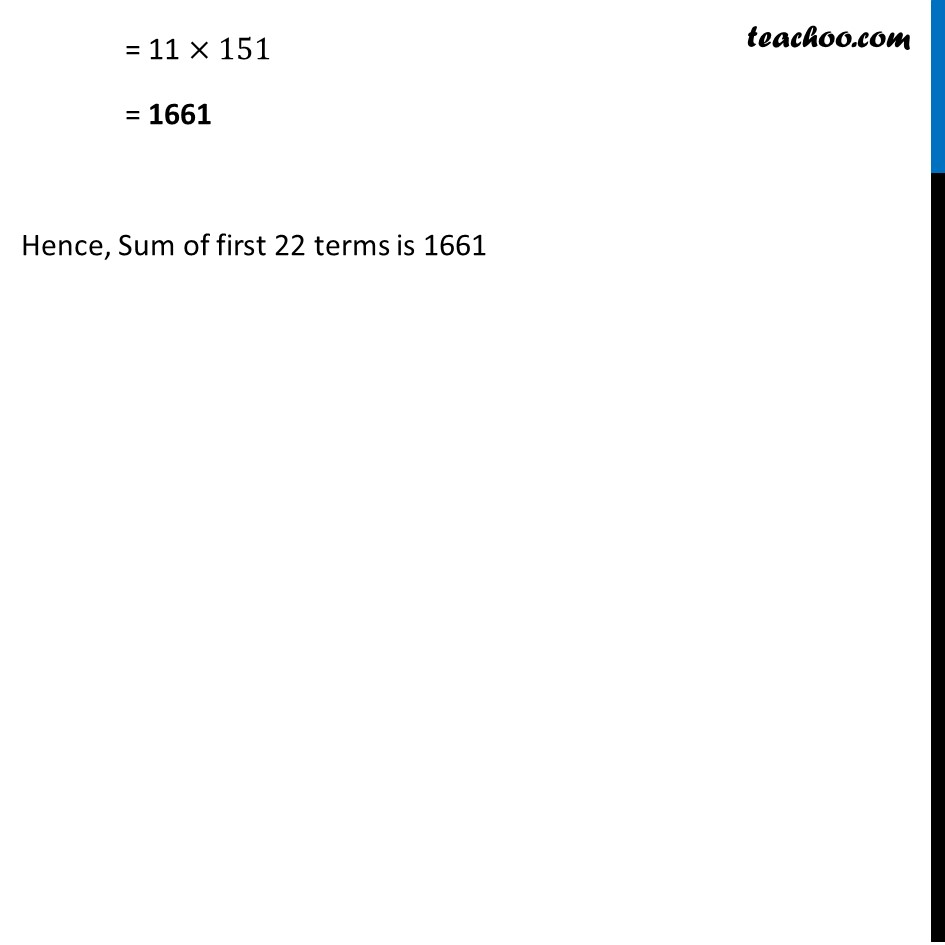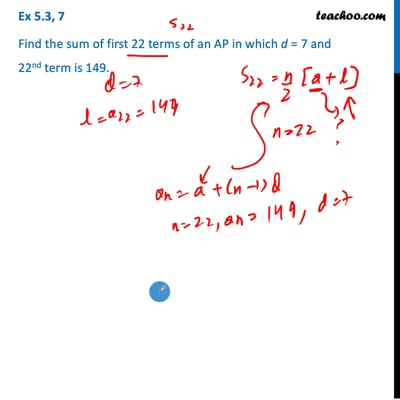Determining AP and finding sum

Chapter 5 Class 10 Arithmetic Progressions (Term 2)
Concept wiseThis video is only available for Teachoo black users

### Transcript

Ex 5.3, 7 Find the sum of first 22 terms of an AP in which d = 7 and 22nd term is 149. Given 22nd term = a22 = 149 Common difference = d = 7 We know that an = a + (n – 1) d Putting n = 22 , d = 7 , an = 149 149 = a + (22 – 1) × 7 149 = a + 21 × 7 149 = a + 147 149 = a + 147 149 – 147 = a 2 = a a = 2 Now, we need to find sum of first 22 terms And we are given a22 = 149 So, last term = l = 149 We use the formula Sn = 𝒏/𝟐 [ a + l ] Putting a = 2, n = 22, l = 149 = 22/2 [2 + 149] = 11 ×151 = 1661 Hence, Sum of first 22 terms is 1661